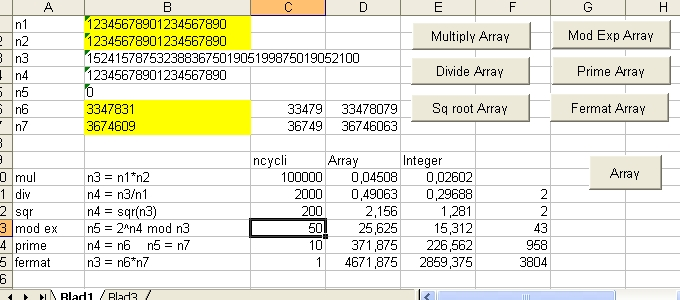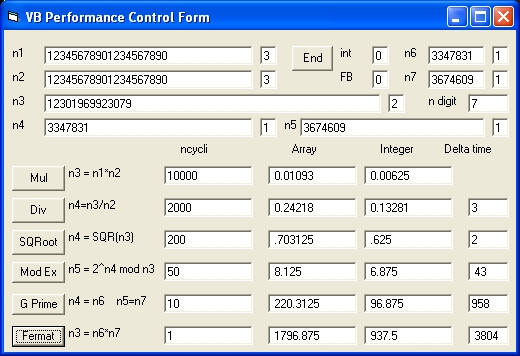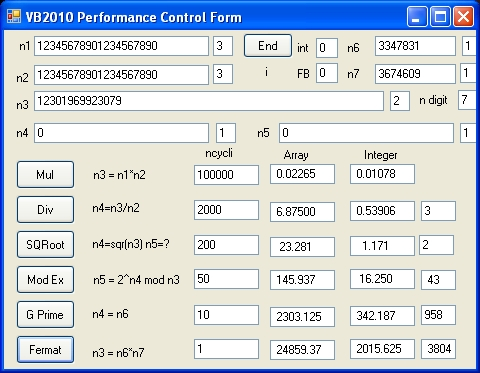## Program "Performance" Benchmark Pentium P4 2.8 and Intel i5 M 460 Visual Basic, Visual Studio 2010 and Excel

The purpose of this document is to evaluate a package of subroutines written in different programming languages necessary to factorize large numbers in two prime numbers
The subroutine package consists of two types of logic: Integer (Array) and (Double Precision) Array
For Integer Array logic the following subroutines are tested:
Mul_Int_Array, Div_int_Array, Sqr_Int_array, GETPRIEM_Int_array and FERMAT_Int_array
For Double Precision Array logic the following subroutines are tested:
Mul_Array, Div_Array, Sqr_array, GETPRIEM_array and FERMAT_array
The three different programming languages are: Excel, Visual Basic 5.0 and Visual Studio 2010.
The two CPU's evaluated are (1) a Pentium P4 and (2) a Intel Core i5 M 460 @ 2.53 Ghz.

The mathematical package is used to factorize large numbers. See: findprim benchmark
To evaluate factorization using Parallel processing select the following link:
Quantum Factoring Performance Evaluation - Using Parallel Processing - VB2010

### General Performance Evaluation - Program "Performance"

The three programs evaluated are: Performance.xls, VB2010_Performance and VB_Performance.

### Excel Basic Benchmark"Blad1" contains 5 Operation Commands:
• Multiply to test multiplication of n1*n2. The result is stored in n3.
• Divide to test division of n3/n1. The result is stored in n4. The remainder in n5
• SQR Root to test Square Root of n3/n1. The result is stored in n4. The remainder in n5
• Prime to test the nearest prime # of n6 and n7. The result of n6 is stored in n4. The result of n7 is stored in n5
• Fermat to test Fermat theorem of n6 and n7. First n6*n7 is calculated and the result is stored in n3. The two prime numbers are stored in n4 and n5
"Blad1" also contains the selection command "Integer" or the command "Array".
• When the "Integer" command is selected the command changes into "Array". The names of the 5 "Operation Commands" also change into "Array"
• When the "Array" command is selected the command changes into "Integer". The names of the 5 "Operation Commands" also change into "Integer"
Pentium P4
 Mul 100000 0.04766 0.02594 Div 2000 0.5 0.28906 SQRoot 200 2.265 1.406 Mod 2 Exp 50 26.25 16.562 Get Prime 10 376.562 229.687 Fermat 1 4671.88 2859.38
Intel Core i5 M 460 @ 2.53 GHZ
 Mul 100000 0.06929 0.06152 Div 2000 0.705 0.560 SQRoot 200 3.046 2.304 Mod 2 Exp 50 x x Get Prime 10 535,156 376,953 Fermat 1 6492,1875 4621,093
Table 1: Performance.xls

What Table 1 shows is that:
• That Pentium P4 is roughly 30% faster than Intel i5 processor.
• That Integer Array logic is faster than Double Precision Array Logic.

### Visual Basic 5.0 BenchmarkOperation of the Control Form is almost identical as the Excel Benchmark
Pentium P4
 Mul 100000 0.01093 0.00625 Div 1000 0.21875 0.13281 SQRoot 100 0.703125 0.625 Mod 2 Exp 50 8.4375 6.5625 Get Prime 20 212.5 96.875 Fermat 1 1765.62 1062.5
Intel Core i5 M 460 @ 2.53 GHZ
 Mul 100000 0,02265 0,01093 Div 1000 0.36328 0.19921 SQRoot 100 1.953125 1.015625 Mod 2 Exp 50 21.5625 10.78125 Get Prime 10 322.6563 153.125 Fermat 1 4140.625 1734.375
Table 2: VB 5.0 Performance

In the above benchmark for the Commands Multiply, Divide and Square Root the numbers n1 = n2 = 12345678901234567890 and n3 = 152415787532388367501905199875019052100 are used
For the Commands Mod Ex, "Get Prime" and Fermat the prime numbers n5 = 3347831 and n6 = 3674609 are used

When you compare the results of Table 1, Table 2 and Table 3:

• That the Pentium P4 is the fastest CPU compared with the Intel Core i5 when only one processor is used.
• That Visual basic 5.0 outperforms Visual Studio 2010.

### Visual Studio VB2010 BenchmarkThe display shows the following functions:
 Mul n1=12345678901234567890. n2= n1=12345678901234567890. n3=n1*n2 = 152415787532388367501905199875019052100 Div n3= 152415787532388367501905199875019052100 n2 =12345678901234567890. n4=n3/n2= 12345678901234567890. n5 = remainder = 0 SQRoot n3= 152415787532388367501905199875019052100 n4=Sqr(n3) = 12345678901234567890. n5 = remainder = 0 Mod Ex n6=3347831 n7= 3674609 n3=n6*n7 =12301969923079 n4=n6=3347831 n5= 2^n4 mod n3 = 3674609
Operation of the Control Form is almost identical as the Excel Benchmark
Pentium P4
 Array Integer Mul 100000 0.02265 0.01078 Div 1000 6.73437 0.53125 SQRoot 100 22.812 1.406 Mod 2 Exp 50 144.687 16.875 Get Prime 1 2248.437 335.937 Fermat 1 25109.37 2031.250
Intel Core i5 M 460 @ 2.53 GHZ
 Array Integer 7 Integer 9 Mul 100000 0.03343 0.00530 0.00520 Div 1000 8.06653 0.5850 0.5600 SQRoot 100 27.170 1.400 2.000 Mod 2 Exp 50 172.201 18.700 18.000 Get Prime 10 2667.903 363.400 353.600 Fermat 1 29188.09 2006.003 1985.003
Table 3: VB2010 Performance

What Table 3 shows is that:
• That Pentium P4 is roughly 30% faster than Intel i5 processor.
• That Integer Array logic is faster than Double Precision Array Logic.
• That Intel i5 processor outperforms Pentium P4 in case of Integer Array Logic

### Subroutine Div_Int_Array

 ```rem = A(In) Div=A(Div) A(Quotient) = zero Do tempin = rem/Div A(temp) = tempin A(Quotient) = A(Quotient)+A(temp) A(in2) = A(Quotient) * A(Div) A(rem) = A(in) - A(in2) rem = A(rem) Loop until tempin < 1 ``` The program uses the following arrays: A(in),A(Div),A(Quotient),A(rem),A(temp) and A(in2) The numbers: rem,Div and tempin are double precision floating point numbers.

### Feedback

None

Created: 21 September 2015